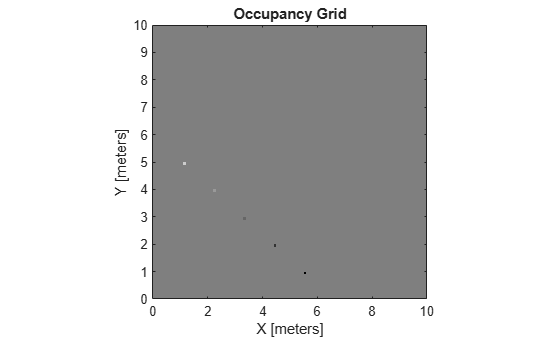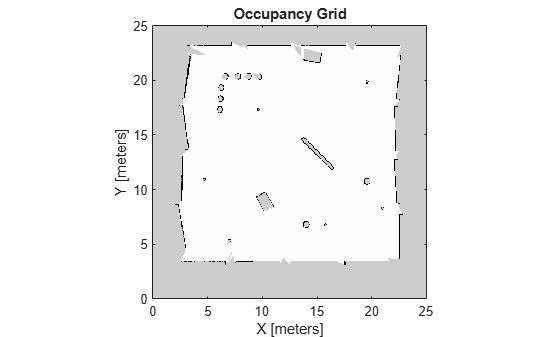# show

Display 2-D occupancy map

Since R2019b

## Syntax

``show(map)``
``show(map,'local')``
``show(map,'grid')``
``show(___,Name,Value)``
``mapImage = show(___)``

## Description

example

````show(map)` displays the occupancy grid `map` in the current axes, with the axes labels representing the world coordinates.```
````show(map,'local')` displays the occupancy grid `map` in the current axes, with the axes labels representing the local coordinates instead of world coordinates.```
````show(map,'grid')` displays the occupancy grid `map` in the current axes, with the axes labels representing the grid coordinates.```
````show(___,Name,Value)` specifies additional options specified by one or more name-value pair arguments.```
````mapImage = show(___)` returns the handle to the image object created by `show`.```

## Examples

collapse all

Create an empty map of 10-by-10 meters in size.

`map = occupancyMap(10,10,10);`

Update the occupancy of specific world locations with new probability values and display the map.

```x = [1.2; 2.3; 3.4; 4.5; 5.6]; y = [5.0; 4.0; 3.0; 2.0; 1.0]; pvalues = [0.2; 0.4; 0.6; 0.8; 1]; updateOccupancy(map,[x y],pvalues) figure show(map)```Inflate the occupied areas by a radius of 0.5 m. The larger occupancy values overwrite the smaller values.

```inflate(map,0.5) figure show(map)```Get the grid locations from the world locations.

`ij = world2grid(map,[x y]);`

Set occupancy values for the grid locations.

```setOccupancy(map,ij,ones(5,1),'grid') figure show(map)```Convert a portable graymap (PGM) file containing a ROS map into an `occupancyMap` for use in MATLAB.

Import the image using `imread`. Crop the image to the playpen area.

```image = imread('playpen_map.pgm'); imageCropped = image(750:1250,750:1250); imshow(imageCropped)```PGM values are expressed from 0 to 255 as `uint8`. Normalize these values by converting the cropped image to `double` and dividing each cell by 255. This image shows obstacles as values close to 0. Subtract the normalized image from 1 to get occupancy values with 1 representing occupied space.

```imageNorm = double(imageCropped)/255; imageOccupancy = 1 - imageNorm;```

Create the `occupancyMap` object using an adjusted map image. The imported map resolution is 20 cells per meter.

```map = occupancyMap(imageOccupancy,20); show(map)```## Input Arguments

collapse all

Map representation, specified as a `occupancyMap` object. This object represents the environment of the vehicle. The object contains a matrix grid with values representing the probability of the occupancy of that cell. Values close to 1 represent a high probability that the cell contains an obstacle. Values close to 0 represent a high probability that the cell is not occupied and obstacle free.

### Name-Value Arguments

Specify optional pairs of arguments as `Name1=Value1,...,NameN=ValueN`, where `Name` is the argument name and `Value` is the corresponding value. Name-value arguments must appear after other arguments, but the order of the pairs does not matter.

Before R2021a, use commas to separate each name and value, and enclose `Name` in quotes.

Example: `'Parent',axHandle`

Axes to plot the map specified as either an `Axes` or `UIAxes` object. See `axes` or `uiaxes`.

Update existing map plot, specified as `0` or `1`. If you previously plotted your map on your figure, set to `1` for a faster update to the figure. This is useful for updating the figure in a loop for fast animations.

## Outputs

collapse all

Map image, specified as an object handle.

## Version History

Introduced in R2019b